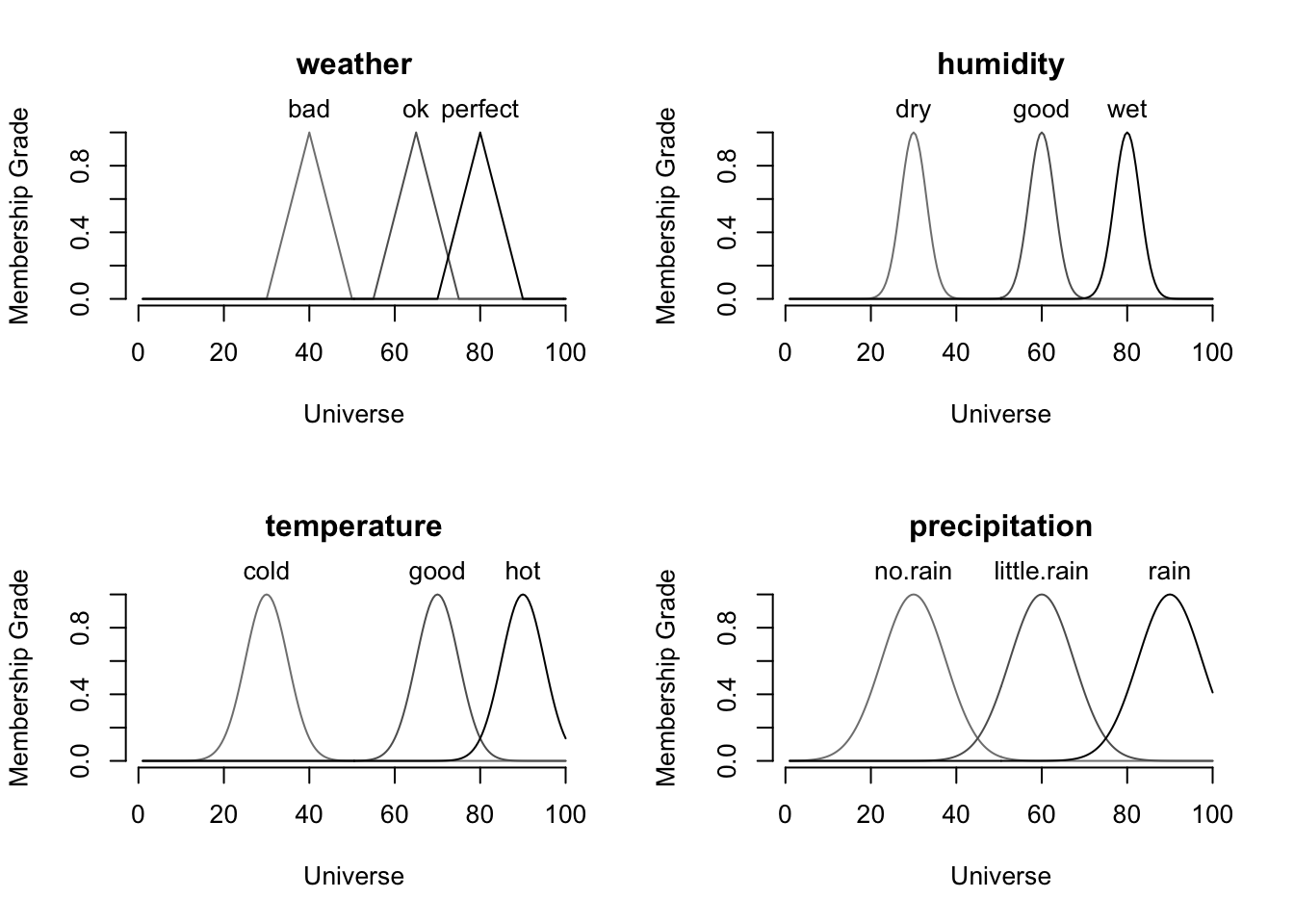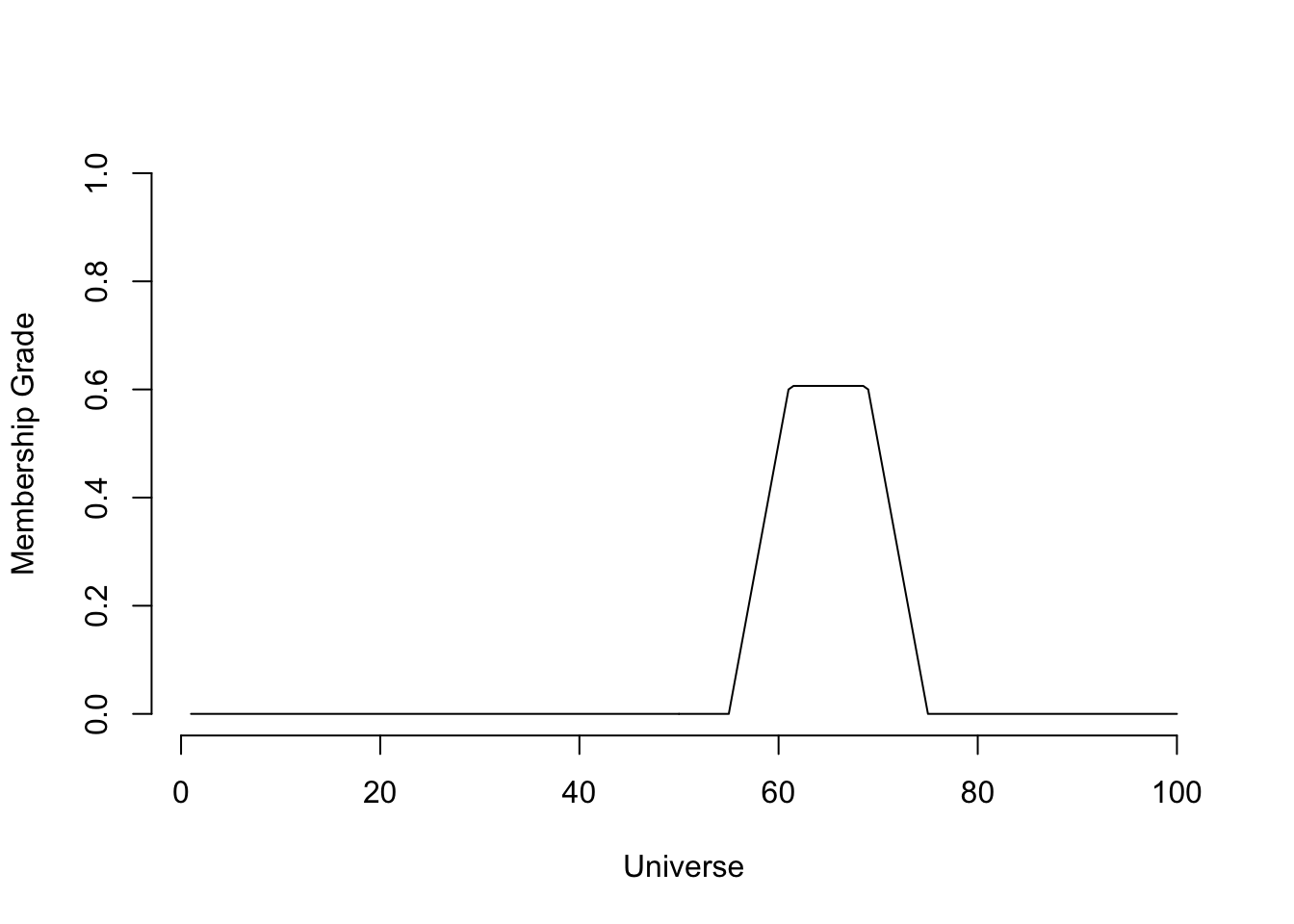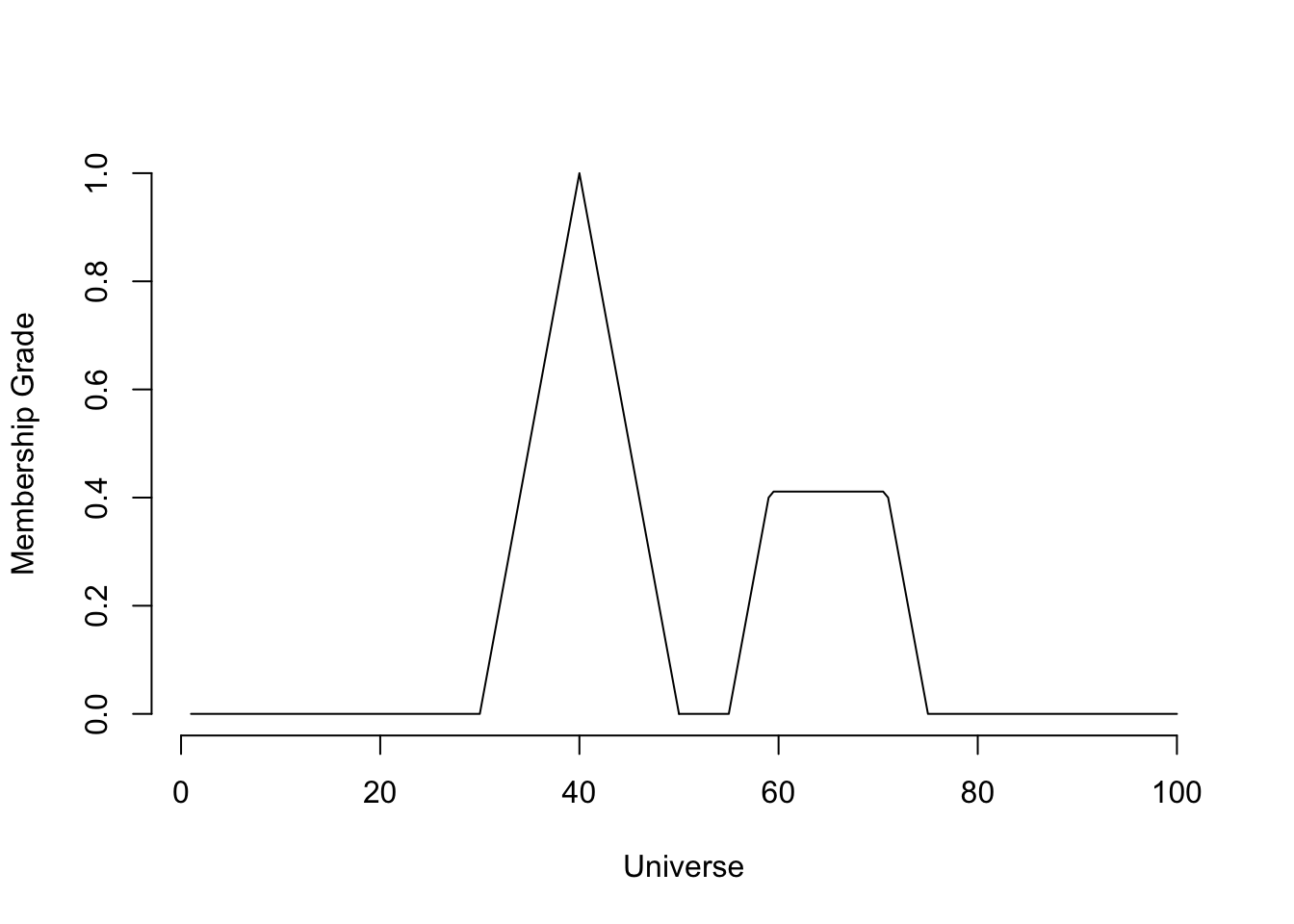## Introduction

In Boolean or two-valued logic, the truth values of a variable will always be either 0 or 1 and in traditional two-valued set theory, an element belongs to a set or not. Similar to this, in a typical classification problem, an observation is classified into one of several different classes. In all of these cases, there is a definitive true value.

Fuzzy logic presents a different approach to these problems. In fuzzy logic, the truth value of a variable or the label (in a classification problem) is a real number between 0 and 1. For example, suppose you are in a pool with a friend. For you, the water is warm and for your friend, the water is cold. After a silly discussion between both of you, you decide to use a thermometer to measure the temperature, and it is 20C. So since both of you know the freezing and boiling point of water (0C and 100C), you said the water is 0.20 hot and your friend is said, it is 0.80 cold. In other words, for you and your friend, there is not an absolute truth about the state of the water.

In this report, I will go through the process of creating a fuzzy system in R. This fuzzy system will use weather as an example and its purpose is to determine how ‘bad’, ‘ok’ or ‘perfect’ the weather is, in other words, the system will assign a score to each of these categories. Keep in mind that in this problem, I will be creating the fuzzy rules based on my opinion of what is a bad/ok/perfect weather; by no means this is something scientific or serious, it is just a guide.

In this tutorial I will use the package “sets” for creating the fuzzy system. For more information about “sets”, check out the official documentation at https://cran.r-project.org/web/packages/sets/sets.pdf

## Set up a simple fuzzy system

The first step in creating a fuzzy system using ‘sets’ is to set the range of the system. The range is a boundary in which all values should belong.

library(sets)
sets_options("universe", seq(1, 100, 0.5))

## The variables

The next step is to define the variables of the fuzzy system. In the previous example of the pool and the temperature, the variables were ‘warm’ and ‘cold’. For this fuzzy system, which is weather-related, the variables I chose are ‘temperature’, ‘humidity’ and ‘precipitation’. So, as mentioned before, the state of the weather (bad, ok or perfect), will be determined according to this variables.

• Temperature
• Humidity
• Precipitation
variables <- set(
temperature = fuzzy_partition(varnames = c(cold = 30, good = 70, hot = 90),
sd = 5.0),
humidity = fuzzy_partition(varnames = c(dry = 30, good = 60, wet = 80),
sd = 3.0),
precipitation = fuzzy_partition(varnames = c(no.rain = 30, little.rain = 60,
rain = 90), sd = 7.5),
weather = fuzzy_partition(varnames = c(bad = 40, ok = 65, perfect = 80),
FUN = fuzzy_cone, radius = 10)
)

You might be wondering what are those values and variables that are inside the parentheses. The answer is that at the time of defining the system, you need to specify the attributes of the variables and give a value to them. For example, the first variable ‘temperature’, has three different attributes or levels: ‘cold’, ‘good’ and ‘hot’, with the values of 30, 70 and 90. This means that if the temperature (using Fahrenheit this time) is 30, then it is ‘cold’, if it 70, it is ‘good’ and if it is 90, it is ‘hot’. For the variables ‘humidity’ and ‘precipitation’ you could read it as “if the humidity percentage is 30, then it is dry” or “there’s a 60% chance that it will rain today”.

The last variable ‘weather’ is the responsible of defining the state of the system. We will see more of this at the end.

## The fuzzy rules

Once the variables are defined, the next step is to define the fuzzy rules of the system. In the previous section, I showed a variable called ‘weather’ that is the final state or the response of the system. The fuzzy rules are the links between the “non-final” variables (temperature, humidity and precipitation) and ‘weather’.

# Fuzzy rules
rules <- set(
fuzzy_rule(temperature %is% good && humidity %is% dry &&
precipitation %is% no.rain, weather %is% perfect),
fuzzy_rule(temperature %is% hot && humidity %is% wet &&
precipitation %is% rain, weather %is% bad),
fuzzy_rule(temperature %is% cold, weather %is% bad),
fuzzy_rule(temperature %is% good || humidity %is% good ||
precipitation %is% little.rain, weather %is% ok),
fuzzy_rule(temperature %is% hot && precipitation %is% little.rain,
weather %is% ok),
fuzzy_rule(temperature %is% hot && humidity %is% dry &&
precipitation %is% little.rain, weather %is% ok)
)

For this system, six rules were defined.

• If the temperature is ‘good’ (refer to the variables to see what ‘good’ is), the humidity is ‘dry’ and the precipitation is ‘no.rain’, then the weather is ‘perfect’.
• If the temperature is ‘hot’, humidity is ‘wet’ and precipitation is ‘rain’, then the weather is ‘bad’.
• If the temperature is ‘cold’, then the weather is ‘bad’.
• If the temperature is ‘good’ or the humidity is ‘good’ or the precipitation is ‘little.rain’, then the weather is ‘ok’.
• If the temperature is ‘hot’ and the precipitation is ‘little.rain’, then the weather is ‘ok’.
• If the temperature is ‘hot’, the humidity is ‘dry’ and precipitation is ‘little.rain’, then the weather is ‘ok’.

Note the && and ||.

Now, let’s build the system.

## The system

model <- fuzzy_system(variables, rules)

The variables and rules of the system.

print(model)
## A fuzzy system consisting of 4 variables and 6 rules.
##
## Variables:
##
## weather(bad, ok, perfect)
## humidity(dry, good, wet)
## temperature(cold, good, hot)
## precipitation(no.rain, little.rain, rain)
##
## Rules:
##
## temperature %is% hot && precipitation %is% little.rain => weather %is% ok
## temperature %is% hot && humidity %is% dry && precipitation %is%  => weather %is% ok
##     little.rain => weather %is% ok
## temperature %is% hot && humidity %is% wet && precipitation %is%  => weather %is% bad
##     rain => weather %is% bad
## temperature %is% good && humidity %is% dry && precipitation %is%  => weather %is% perfect
##     no.rain => weather %is% perfect
## temperature %is% good || humidity %is% good || precipitation %is%  => weather %is% ok
##     little.rain => weather %is% ok
## temperature %is% cold => weather %is% bad

This is the plot of the system.

plot(model)As you can see, some of the plots present overlapping between the properties of the variables. For example, in the temperature plot, there is overlapping between ‘good’ and ‘hot’, so if the temperature is 80, then it is around 0.15 ‘good’, 0.15 ‘hot’ and 0.0 ‘cold’.

## Examples

These are some examples done to test the system.

#### Temperature = 75, humidity = 0 and precipitation = 70

example.1 <- fuzzy_inference(model, list(temperature = 75, humidity = 0,
precipitation = 70))

Now, we defuzzify the example to transform the parameters into an actual number.

gset_defuzzify(example.1, "centroid")
##  65
plot(example.1)So, according to the system, the weather is 0.6 ok (see the weather plot)

#### Temperature = 30, humidity = 0 and precipitation = 70

The next example, is very similar to the previous one. The only difference is the temperature parameter, which now is 30. What do you think is going to happen?

example.2 <- fuzzy_inference(model, list(temperature = 30, humidity = 0,
precipitation = 70))
gset_defuzzify(example.2, "largestofmax")
##  40
plot(example.2)sets_options("universe", NULL)  # Reset the universe

By lowering the temperature, the model lowers the amount of ‘ok’ weather to around 0.4 and creates a new ?smoothpeak with global maxima at 40, meaning that the weather is 1.0 ‘bad’.

I did a little cheating to get this result. If you look at the variables and the rules, you can see that a temperature of 30 is ‘cold’ and that there is a rule stating that if the temperature is ‘cold’, then the weather is ‘bad’.

## Closure

In this tutorial, I introduced the basic of fuzzy logic and presented an example using R. If you are interested in learning more about this topic and how to apply it using R, I recommend the official documentation of the ‘sets’ package linked at the beginning. Also, the Wikipedia page about fuzzy logic is pretty good (https://en.wikipedia.org/wiki/Fuzzy_logic).